# Julia set

G. Julia [a1] and P. Fatou

studied the iteration of rational mappings. Letdenote the-fold composite of the functionwith itself. A point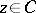is an element of the so-called Fatou set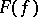ofif there exists a neighbourhoodofinsuch that the family of iterates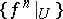is a normal family. The Julia setis the complement of the Fatou set.has the following properties: 1)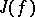is non-empty and perfect (cf. Perfect set); 2)equals the closure of the set of repelling periodic points (cf. Periodic point); 3)is either totally disconnected (cf. Totally-disconnected space) or connected by Jordan arcs or coincides with; 4)is invariant with respect toand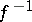; and 5)is an attractor (cf. Strange attractor) of the inverse iterated mapping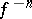. In almost-all caseshas a fractal dimension and may be termed a fractal (cf. Fractals). D. Sullivan has given an exhaustive classification of the elements of the Fatou set with respect to their dynamics. Every component ofis either periodic or pre-periodic. Letbe such a periodic domain and letbe its period. Writing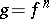one has the following five kinds of dynamics:

a)is an attracting domain;contains an attracting periodic pointwith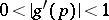.

b)is a super-attractive domain;contains a periodic pointwhich is also a critical point, i.e.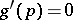.

c)is a parabolic domain; its boundary contains a periodic pointwith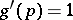.

d)is a Siegel disc (cf. Siegel domain);is simply connected andis analytically equivalent to a rotation.

e)is a Herman ring:is conformally equivalent to an annulus andis analytically conjugate to a rigid rotation of an annulus.

Here a pre-periodic point is a point some iterate of which is periodic. A fixed pointofis super-attractive if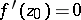. (Recall that if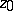is a fixed point, thenis attractive if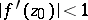and repelling if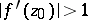.)

The existence of Herman rings has been proved, but they have never yet (1989) been observed.

The best studied case is the quadratic mapping. All phenomena are present there, with the exception of a Herman ring. Allfor whichis connected form the Mandelbrot set, the bifurcation diagram ofin the parameter space of. See also Chaos; Routes to chaos.